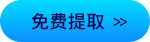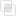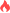python使用choice生成随机数

Python2021-04-26 15:14:07899浏览 · 0收藏 · 0评论1、概念

2、语法

`choice(a, size=None, replace=True, p=None)`

3、参数

4、实例

```# 以数组形式
import numpy as np
import pandas as pd

RandomNumber=np.random.choice([1,2,3,4,5],\
size=100, replace=True,\
p=[0.1,0.1,0.3,0.3,0.2])
pd.Series(RandomNumber).value_counts() # 计算频数分布value_counts()函数
pd.Series(RandomNumber).value_counts()/100  #计算概率分布```python关键字参数的多种使用python函数接收不同类型的参数python函数返回多个返回值python类方法如何定义python类属性的使用注意python静态方法如何定义python特殊方法有哪些python类的继承如何定义？python类的继承链分析python爬虫如何获取ip代理池？python常见过滤器的整理java懒惰评估如何实现1026

java尾部递归如何使用687

python如何判断文件夹内的重复图片1077